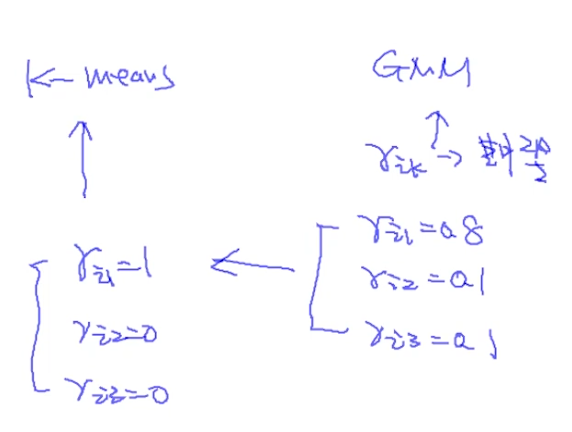# Graphical Model和Latent Variable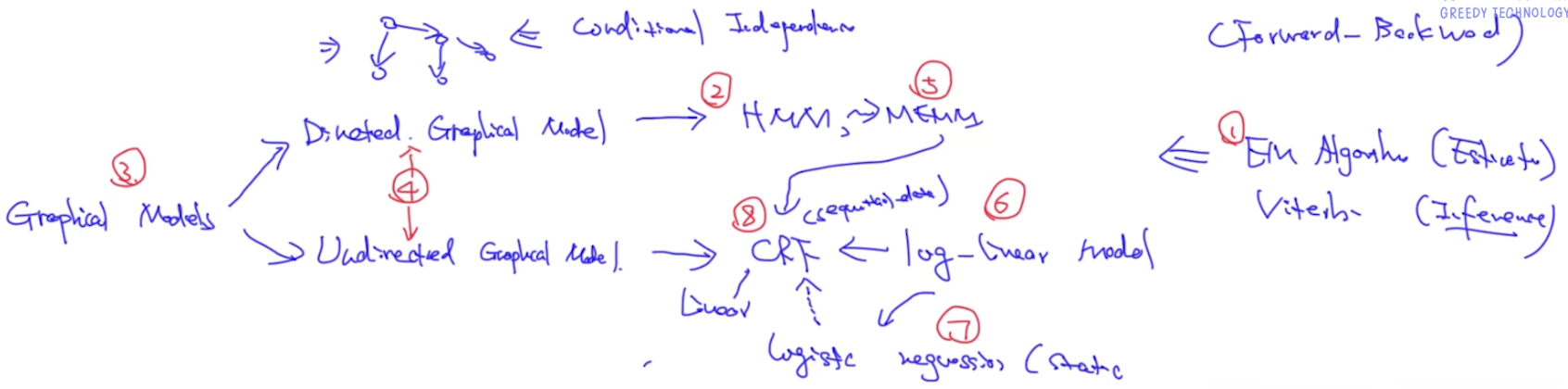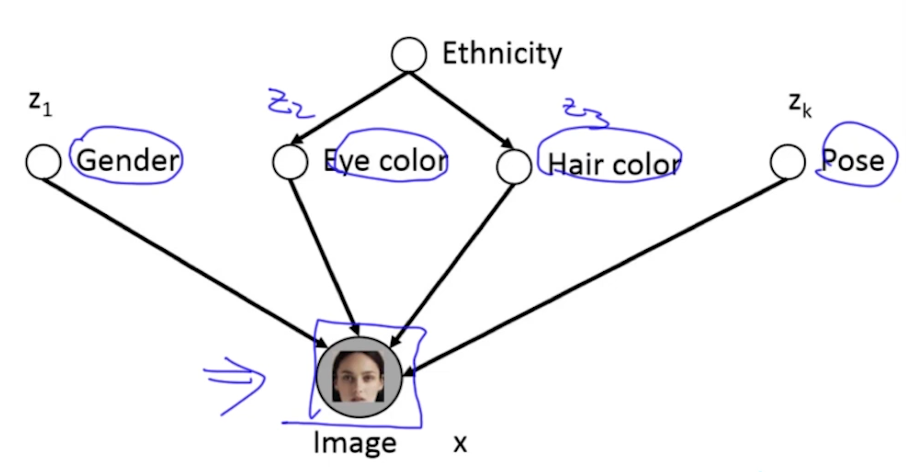EM的核心是计算参数theta，如下图：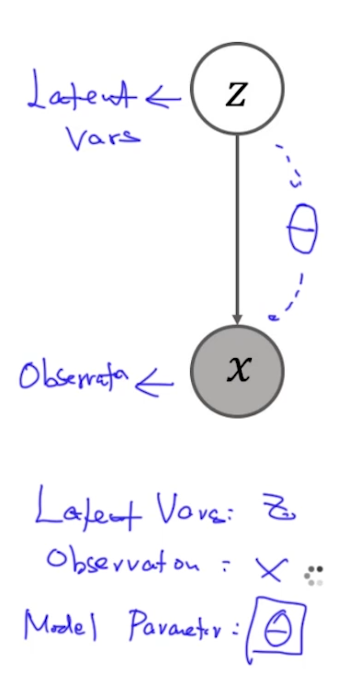• Complete case：(z, x)都是可观测的，如逻辑回归（已知x预测$\theta​$），一般使用MLE
• Imcomplete case：x是可观测的，z是不可观测的，使用EM style算法

# MLE for Complete and Imcomplete Case

【注】：在图模型中联合概率很难表示，有几个技巧可以尝试：
（1）使用conditional independence对形如$P(a,b,c,d,e|\theta)$进行转换：例如n-gram语言模型P(I hate ad|y=垃圾)，在朴素贝叶斯中就会近似为P(I|y=垃圾)P(hate|y=垃圾)P(ad|y=垃圾)
（2）使用D-seperation或Markov Blanket对形如$P(a|b,c,d,e,f)$进行转换：比如发现d、e、f对a没有影响，转换为P(a|b,c)，进而再转化为函数形式f(\theta)，再使用优化方法找出解或者近似解
（3）在Incomplete Case中，由于z也是未知的，所以把z边缘化了
（4）上面图中$p(z|\theta)$和$p(x|z, \theta)$也可以认为是两个函数$f(\theta)和g(\theta)$，也可以使用优化算法进行求解。对于Incomplete Case，$f(\theta)和g(\theta)$很难求解，所以使用EM-style算法求解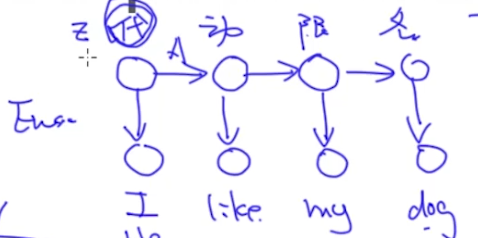# EM Derivation

EM中一共有3类变量：

• $\theta​$: model parameter
• $z​$: latent variable
• $x$: obversation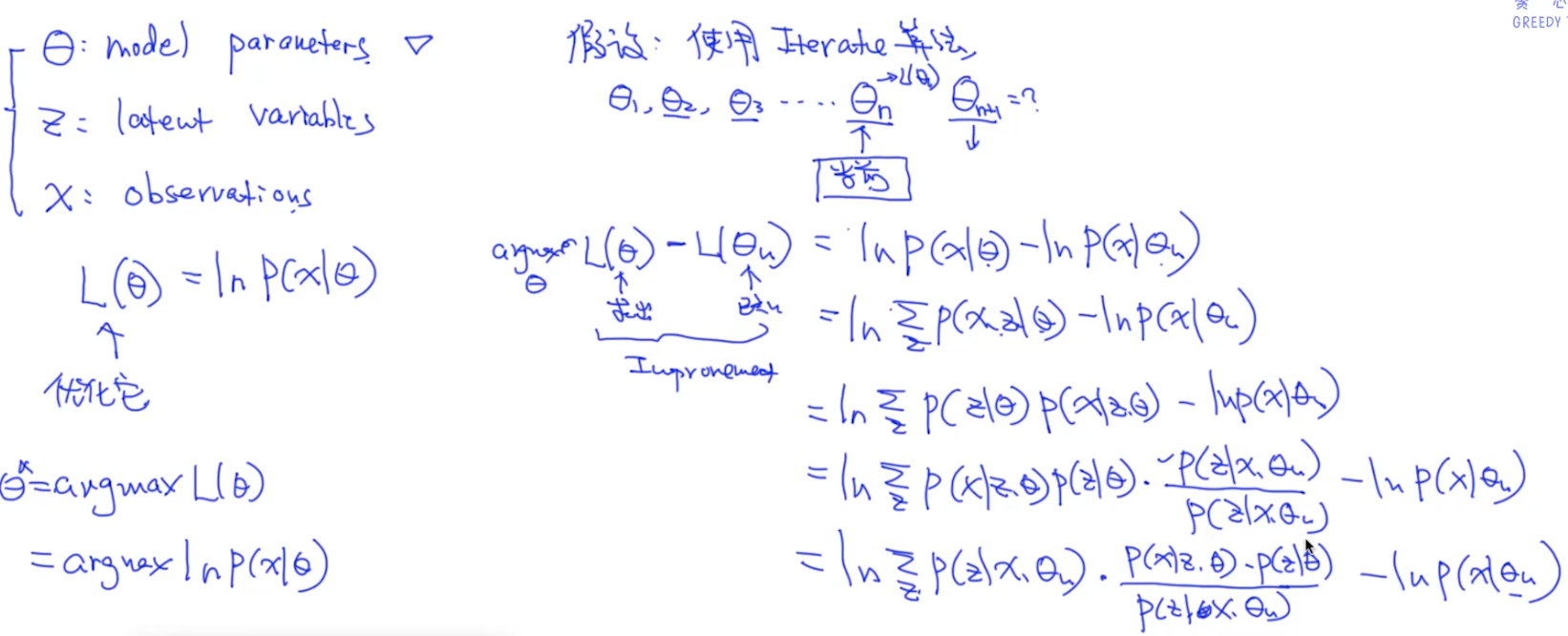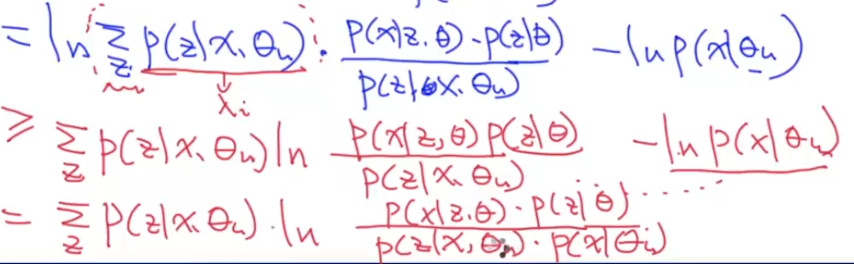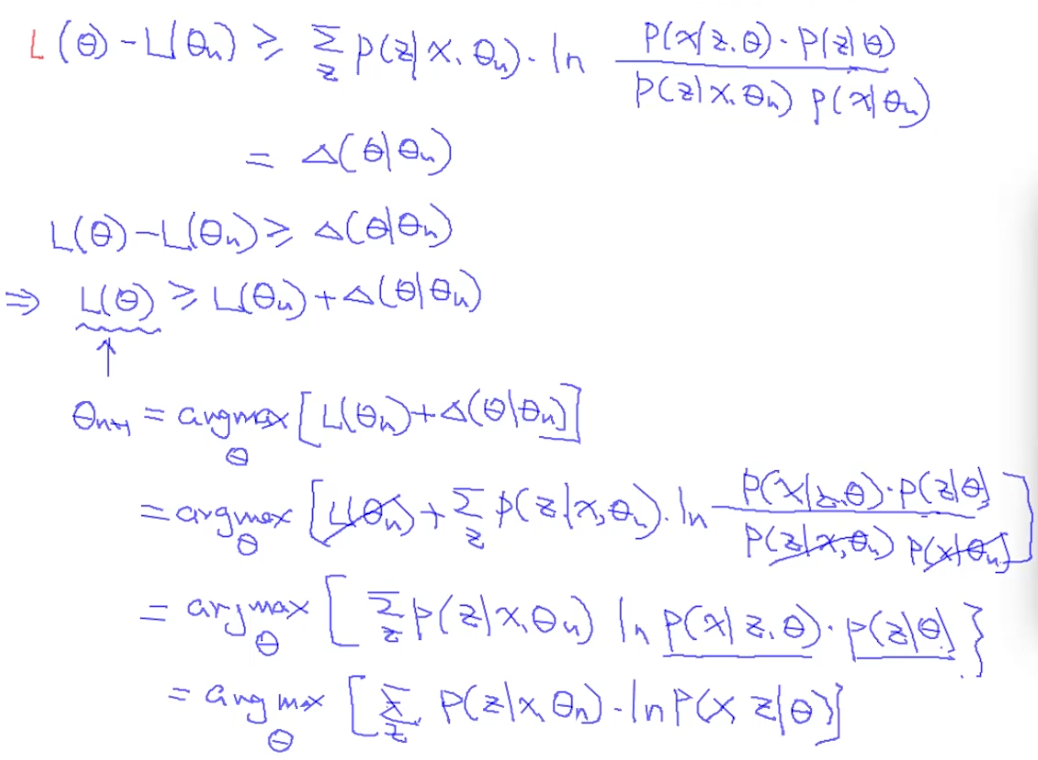【注】：你可能想问，已知$x$, $\theta_n$，是怎么求出$P(z|x, \theta_n)$的呢？在具体问题中，我们通常会把$P(z|x, \theta_n)$写成一个函数的形式，自然就可以求出来了

EM算法其实就是两个步骤的一个循环，已知$x$, $\theta$求$z$的期望，再用这个$z$求解$\theta$，再去迭代得求解$z$，如下图：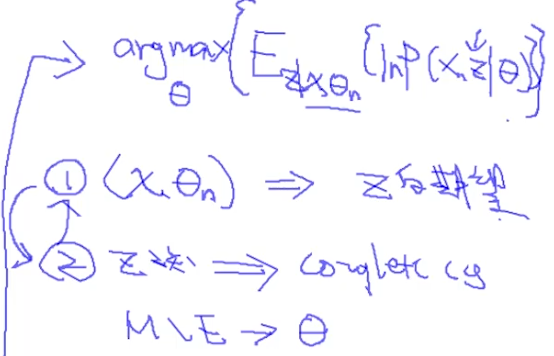EM算法一定是收敛的，它本质上是coordinate descent，即按照坐标轴去优化。

# K-means

K-means整体上就是选中心点—>根据与中心点的举例聚类—>重新计算中心点，这样一个迭代的过程，如下图所示：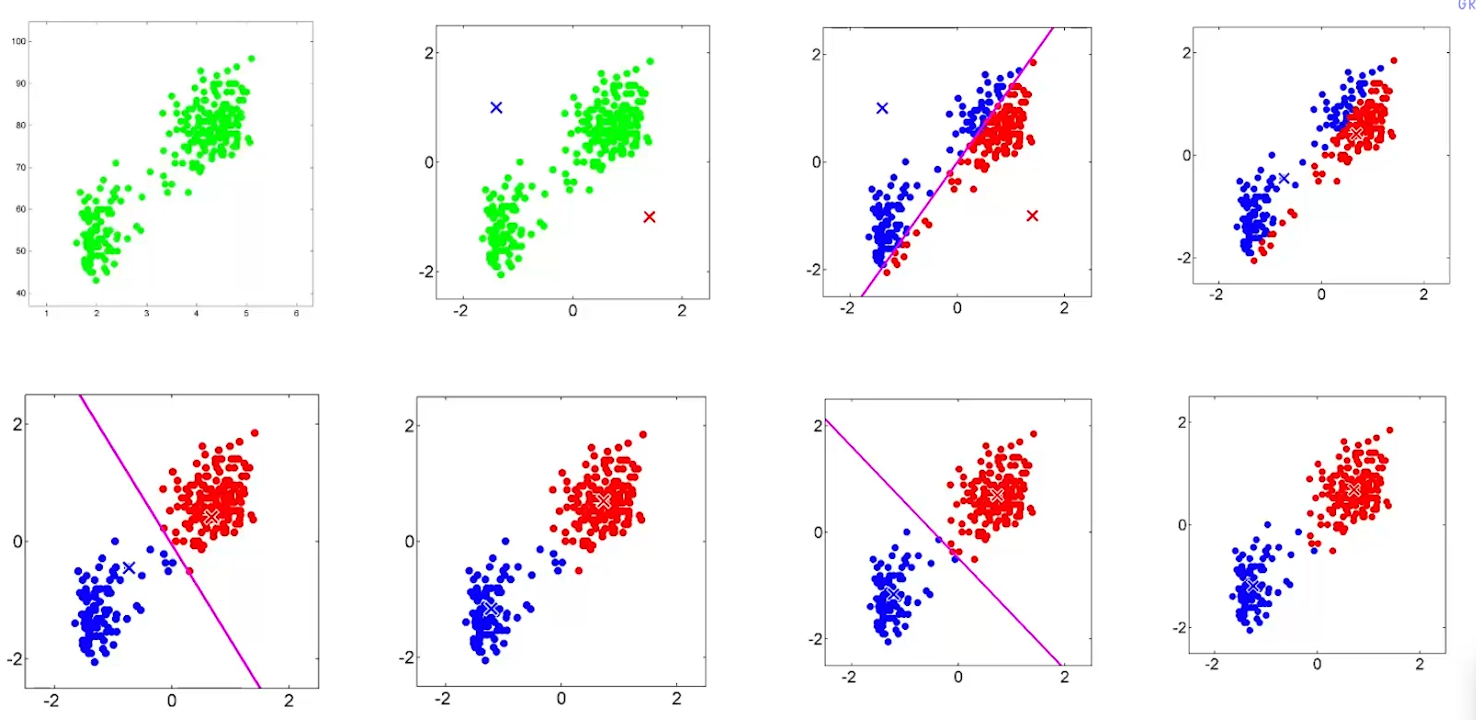## Cost Function

Kmeans的代价函数如下图：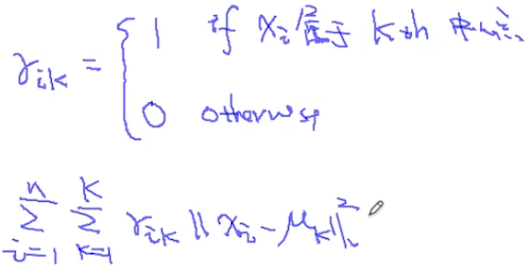• $\mu_k$表示中心点，可以看成是模型参数
• $\gamma_{ik}$表示对$\mu_k$的选择，可以看成是隐变量

## K-means和EM的关系

K-means的迭代过程就是先选择$\mu_k$，再计算$\gamma_{ik}$，再根据聚成的簇重新选择$\mu_k$。但K-means是一个EM-style的算法，而不是一个严格意义的EM。因为在EM中，要计算的是隐变量的期望值，即对于$\gamma_{ik}$表示$x_i$属于第$k$个cluster的概率，则$\gamma_{i0}=0.8$, $\gamma_{i1}=0.2$….（有点像GMM哈）。但在K-means中，我们直接令$\gamma_{i0}=1$了。因此Kmeans也叫Hard-Clustering，GMM也叫Soft-Clustering。如下图：